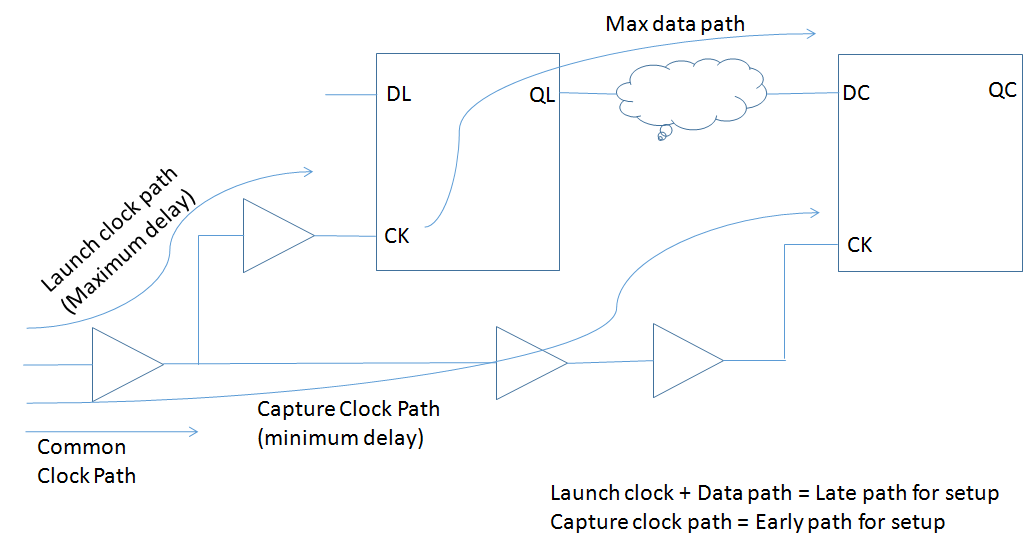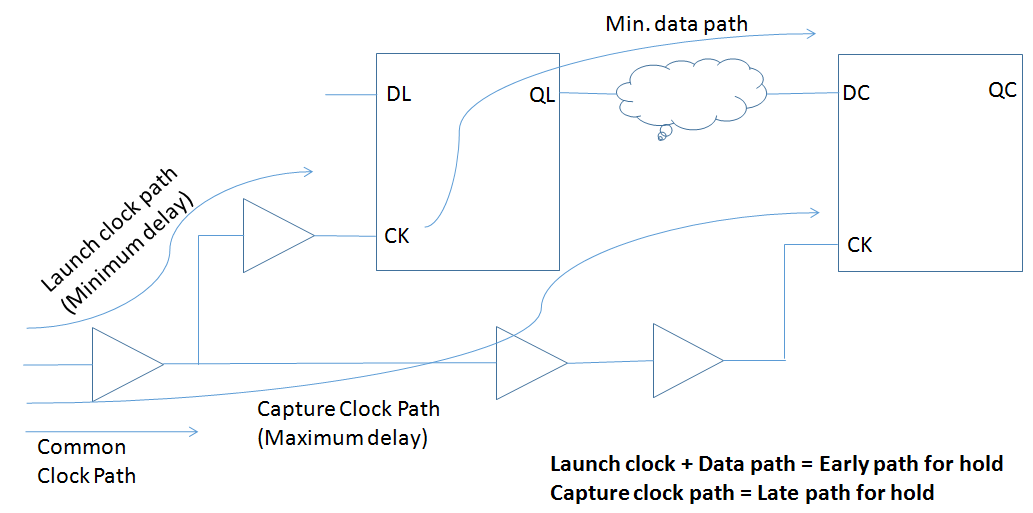## Wednesday, March 23, 2016

### OCV and AOCV (Advanced On-Chip Variations)

In this article i will try to cover OCV (On-Chip variations) concepts emphasizing on AOCV (Advanced OCV). You might have used OCV extensively during the timing closure. We have heard about AOCV but a little is known behind the concept of AOCV. Let's explore OCV and AOCV in a way in which one can easily understand.

While fabricating a chip, we take a wafer/die and start our process. Let's start with a question, If you are putting the same chip (design) in different portions of die, will the chip behavior be same (timing wise)? The answer is it can be or can't be but mostly NO, this is the reason where on-chip variations plays a role. Different portions of design may also see different power supply voltage and temperature. It is possible that two regions of the same chip may not encounter the identical PVT conditions. This is due to the fact that environmental and process parameters are not uniform across the die area. Due to these variations MOS characteristics will change.

You can describe on-chip variation using a random and a deterministic component. The random component of critical parameter variation occurs from lot to lot, wafer to wafer, and die to die. Examples are variations in gate-oxide thickness, implant doses, and metal or dielectric thickness. The deterministic component comprises variations that you can predict from the location on the wafer or the nature of surrounding patterns. These variations relate to proximity effects, density effects, and the relative distance of devices. Examples are variations in gate length or width and interconnect width. While working in deep-submicron processes, designers can no longer ignore the variation between devices and interconnect characteristics on the same die.

Modelling OCV :
Because of the above said variations, these can affect the wire delays and cell delays in different portions of the chip. To account for this local PVT variations one need to incorporate OCV analysis during STA. Since the clock and data paths can be affected differently by the OCV, the timing verification can model the OCV effect by making the PVT conditions for the launch and capture paths to be slightly different. In STA one can model these variations by derating the delays of specific paths i.e by making path slower or faster as per the need. The cell delay as well as net delay can be derated to model OCV.

OCV derating for Setup Check:
While checking for the setup check due to OCV, the worst condition is when the launch clock path and data path OCV condition result into largest delay while the capture clock path has the OCV conditions which results in smallest delays. Here these largest and smallest delays are to account for local PVT variations within die and this case is the most restrictive one for setup check. Following figure will give you an overview about the late/early path for setup check in case of OCV.Now in order to model these late/early paths we used to provide the following constraint,
set_timing_derate -early 0.9
set_timing_derate -late 1.1
The above constraint will derate the minimum/early paths by -10% (i.e the path will be made more faster) and derate the maximum/late paths by 10% (i.e the paths will be delayed more). As we can see from the above figure that for setup check the launch clock and data path, which is maximum/late path for setup check, will be multiplied by derating factor with -late option. On the other hand capture clock which is minimum/early path for setup check, will be multiplied by derating factor with -early option. If no derating factors applied then derating factor of 1.0 is assumed.

Note: We can also derate only net delay or cell delay depending on the scenario using -net_delay or -cell_delay switch with set_timing_derate.

OCV derating for Hold Check:
While checking for the hold check due to OCV, the worst condition is when the capture clock path OCV condition result into largest delay while the launch clock and data path has the OCV conditions which results in smallest delays. Following figure will give you an overview about the late/early path for hold check in case of OCV.Similar to the setup check, if we apply the following constraint then,
set_timing_derate -early 0.9
set_timing_derate -late 1.1
The above constraint will derate the minimum/early paths by -10% (i.e the path will be made more faster) and derate the maximum/late paths by 10% (i.e the paths will be delayed more). As we can see from the above figure that for hold check the launch clock and data path, which is minimum/early path for hold check, will be multiplied by derating factor with -early option. On the other hand capture clock which is maximum/late path for hold check, will be multiplied by derating factor with -late option. If no derating factors applied then derating factor of 1.0 is assumed.

Note: We can also derate only net delay or cell delay depending on the scenario using -net_delay or -cell_delay switch with set_timing_derate.With -cell_check option even we can derate the setup/hold value of a cell.

AOCV:
As we have seen till now the concept of OCV is taken care by applying a fixed derating factor. This is a little bit pessimistic. As the technology node is shrinking and the frequency is increasing, the timing closure is becoming much difficult so we can't afford pessimism in the design. To overcome this pessimism we use the concept of Advanced-OCV. In AOCV we don't apply fixed derating factor, derating factor is decided based on the location and depth of the logic.

Distance based AOCV is used to model global variation effects. It is assumed that different parts of the chip will vary more so that's why as distance increases you see more variations. Let's suppose i have divided a chip into four parts - part1, part2, part3 and part4. Then the variation between part1 and part3 or part1 and part4 or part1 and part2 will be more. so in AOCV derating factor increases as the distance increases.
Depth based AOCV is used to model local variation effects. Local variations tend to cancel out over the course of long path as some delays are little worse, some are little better. so in general we say that as depth increases the derating factor decreases in case of AOCV.

AOCV differs from traditional fixed derates (OCV) in the way that derate tables for each cell are indexed by path depth. The AOCV tables include the information so that a different derate value is chosen for a cell at the begining of a path Vs the same cell near the end of the path.

Let's assume that there are 6 buffers in a data path. Generally in OCV, the cell delays are derated with the same number e.g 10ps. So 10ps is worst delay for each cell. Therefore total worst delay is 10x6=60ps. This value is used for slack calculation in timing analysis. But the fact is that all the cells won't produce 10ps delay as worst delay. Some of the cells can be fast also. so the accumulative delay will be less than 60ps. This probability of less delay (than worst) increases as the depth (no. of cells) increases.

When applied during timing analysis a cell in a short path will use a different derate factor than that same cell found in a long path. By allowing different derate for every cells, in each different path stage based OCV eliminates pessimism in a fixed derate strategy OCV. Stage based OCV derates can be computed using foundry models, LPE netlists and liberty timing libraries. The models and SPICE netlists help to define the cells' sensitivity to variation while the timing libraries can be used to identify timing arcs and desired ranges for input slew and ouput load. Derating tables for individual cells involves statistically simulation of these input conditions and timing arcs and combining the results in to final derate values.

## Sunday, March 13, 2016

### Basics of Setup and Hold Part - 3

Previous Page>>

Q.1 >> Find
1. Is there any setup violation in following flop network ?
2. Maximum frequency at which following flop network work fine ?

T = 100ns (10Mhz)
Tc2q = 10ns
Tpd = 50ns
Ts = 5ns
ANS >>
There is no setup time violation if
Tc2q + Tpd < T - Ts
Tc2q + Tpd = 10 + 50 = 60 ns
T - Ts = 100 - 5 = 95 ns
So 60 < 95 (TRUE)
There is no setup violation.
So to find out maximum frequency or minimum time period at which network work is.
Tc2q + Tpd = Tmin - Ts
10 +  50 =   T  -  5
T = 50 + 10 + 5   =   65ns
Fmax  = (1000/65) Mhz =   15.38Mhz
--------------------------------------------------------------------------------------------------------------
--------------------------------------------------------------------------------------------------------------
Q.2 >> Find
1. Is there any setup and hold violation ?
2. Maximum frequency at which it work fine ?
1. To check hold violation in network we take worst hold conditions like -  This network work fine for all condition if maximum clock arrival time is less than minimum data arrival time.

Data Path : D1(min) + D2(min) + Tc2q(min) + Tpd(min)
Minimum Data Arrival Time :  3 + 3 + 8 + 6 = 20ns

Clock Path : D1(max) + D3(max) + D4(max) + Th
Maximum Data requested Time :  6 + 6 + 6 + 2 = 20ns

Slack = Data Arrival time - Data Requested Time
= 20 - 20 = 0ns

Fortunately, This meet Slack and network will work under this condition.

1. To check setup violation in network we take worst setup conditions like -  This network work fine for all condition if maximum data arrival time is less than minimum clock arrival time.

Data Path : D1(max) + D2(max) + Tc2q(max) + Tpd(max)
Maximum Data Arrival Time :  6 + 6 + 12 + 9 = 33ns

Clock Path : D1(min) + D3(min) + D4(min) + Tclk - Ts
Minimum Clock Arrival Time :  3 + 3 + 3 + 25 - 5 = 29ns
(Minimum Data requested Time)
Slack = Data requested time - Data arrival time
= 29 - 33
= -4ns

This is HUGE neg. Slack and network will not work under this condition. Now maximum frequency at which network work fine is (Tmin) from following setup timing equation.
D1(max) + D2(max) + Tc2q + Tpd(max) = D1(min) + D3(min) + D4(min) + Tmin - Ts

6 + 6 + 12 + 9 = 3 + 3 + 3 + Tmin - 5
Tmin = 33 - 4 = 29ns
Fmax = (1000/29) Mhz = 34.4Mhz
--------------------------------------------------------------------------------------------------------------
--------------------------------------------------------------------------------------------------------------